# High School Math : How to find the perimeter of an acute / obtuse triangle

## Example Questions

### Example Question #173 : Plane Geometry

Two similiar triangles have a ratio of perimeters of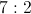.

If the smaller triangle has sides of 3, 7, and 5, what is the perimeter of the larger triangle.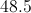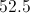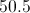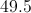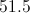Explanation:

Adding the sides gives a perimeter of 15 for the smaller triangle. Multipying by the given ratio of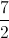, yields 52.5.

### Example Question #1 : Triangles

Two sides of an isosceles triangle are 20 and 30. What is the difference of the largest and the smallest possible perimeters?

0

10

30

15

10

Explanation:

The trick here is that we don't know which is the repeated side. Our possible triangles are therefore 20 + 20 + 30 = 70 or 30 + 30 + 20 = 80.  The difference is therefore 80 – 70 or 10.

### Example Question #174 : Plane Geometry

Two similiar triangles exist where the ratio of perimeters is 4:5 for the smaller to the larger triangle. If the larger triangle has sides of 6, 7, and 12 inches, what is the perimeter, in inches, of the smaller triangle?

18

25

23

20

20

Explanation:

The larger triangle has a perimeter of 25 inches. Therefore, using a 4:5 ratio, the smaller triangle's perimeter will be 20 inches.

### Example Question #131 : Geometry

If a = 7 and b = 4, which of the following could be the perimeter of the triangle?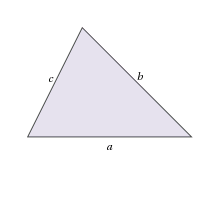I. 11

II. 15

III. 25

I and II Only

II and III Only

I, II and III

I Only

II Only

II Only

Explanation:

Consider the perimeter of a triangle:

P = a + b + c

Since we know a and b, we can find c.

In I:

11 = 7 + 4 + c

11 = 11 + c

c = 0

Note that if c = 0, the shape is no longer a trial. Thus, we can eliminate I.

In II:

15 = 7 + 4 + c

15 = 11 + c

c = 4.

This is plausible given that the other sides are 7 and 4.

In III:

25 = 7 + 4 + c

25 = 11 + c

c = 14.

It is not possible for one side of a triangle to be greater than the sum of both of the other sides, so eliminate III.

Thus we are left with only II.